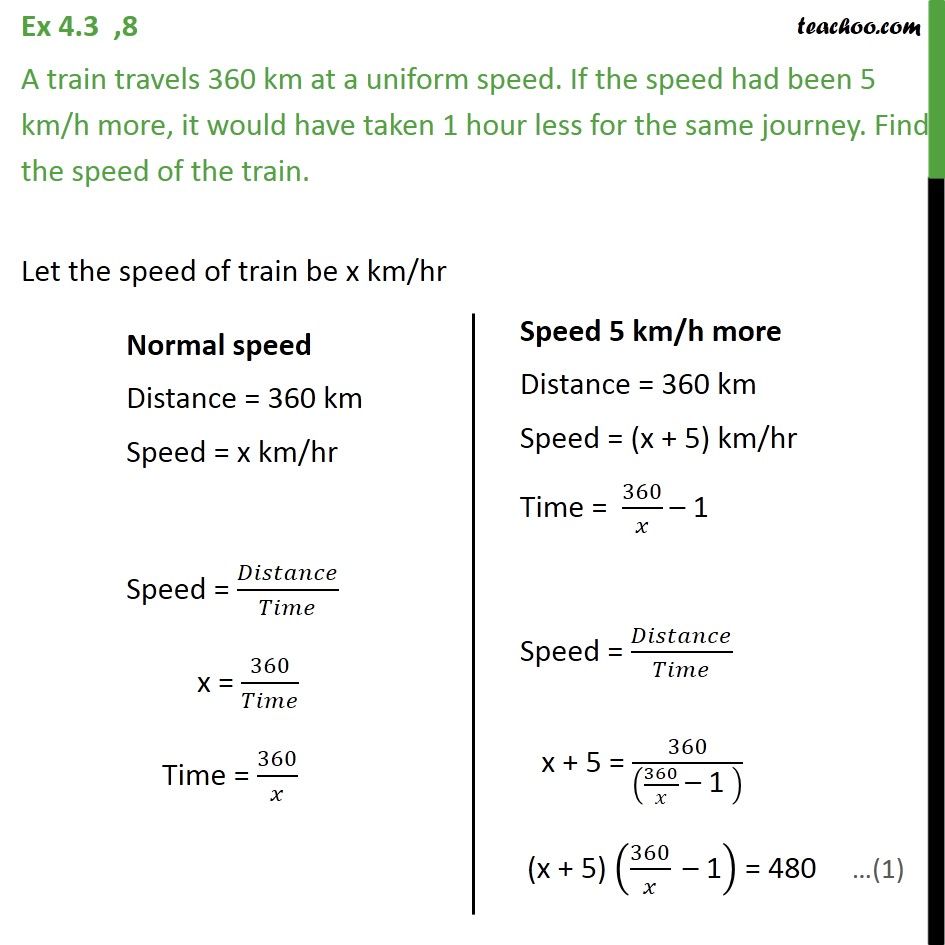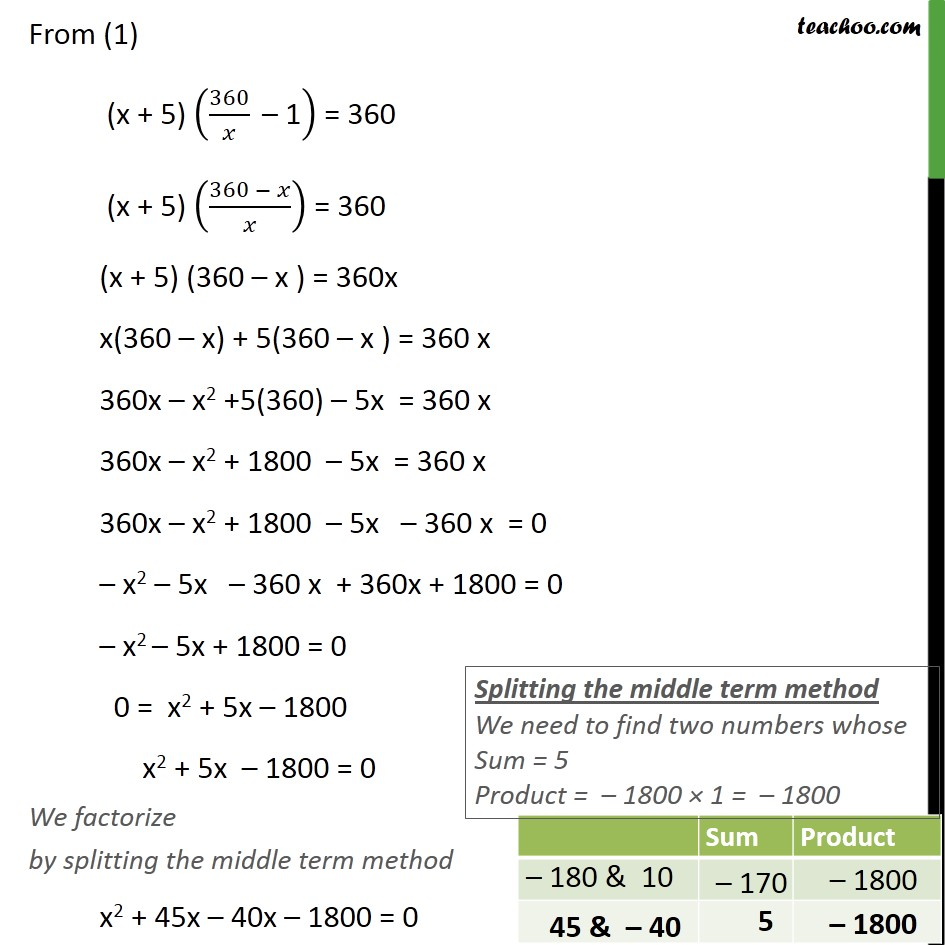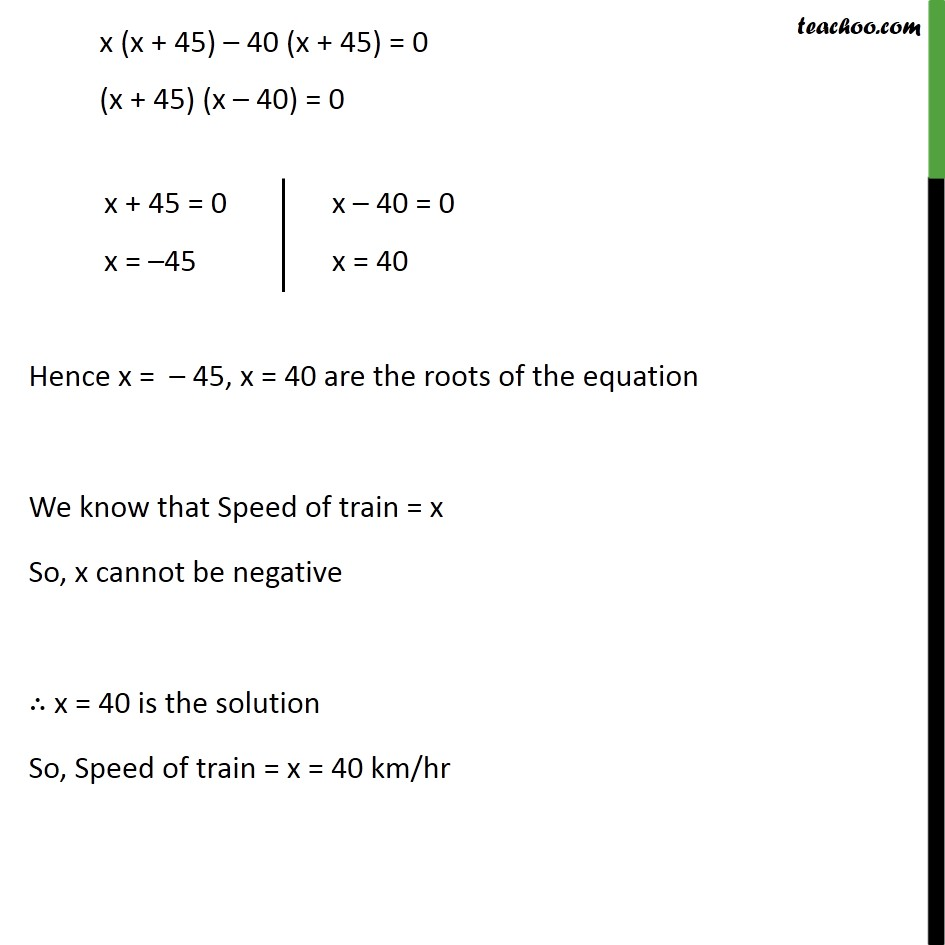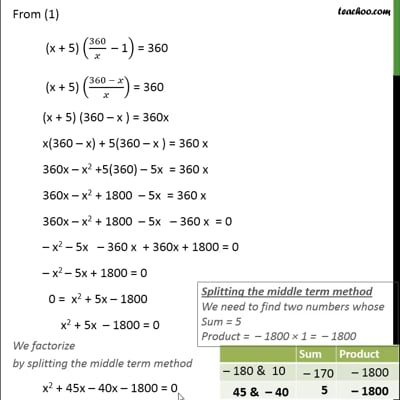Chapter 4 Class 10 Quadratic Equations

Class 10
Important Questions for Exam - Class 10This video is only available for Teachoo black users

Introducing your new favourite teacher - Teachoo Black, at only ₹83 per month

### Transcript

Ex 4.3 ,8 A train travels 360 km at a uniform speed. If the speed had been 5 km/h more, it would have taken 1 hour less for the same journey. Find the speed of the train. Let the speed of train be x km/hr From (1) (x + 5) (360/ " 1" ) = 360 (x + 5) ((360 )/ ) = 360 (x + 5) (360 x ) = 360x x(360 x) + 5(360 x ) = 360 x 360x x2 +5(360) 5x = 360 x 360x x2 + 1800 5x = 360 x 360x x2 + 1800 5x 360 x = 0 x2 5x 360 x + 360x + 1800 = 0 x2 5x + 1800 = 0 0 = x2 + 5x 1800 x2 + 5x 1800 = 0 We factorize by splitting the middle term method x2 + 45x 40x 1800 = 0 x (x + 45) 40 (x + 45) = 0 (x + 45) (x 40) = 0 Hence x = 45, x = 40 are the roots of the equation We know that Speed of train = x So, x cannot be negative x = 40 is the solution So, Speed of train = x = 40 km/hr